INTRODUCTION TO VECTORS - PART 1

Example 1:

Find the component form of a vector with initial point P at (1, 2) and terminal point Q at (4, 4). Name the new vector v.

Given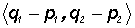and Pand Q, we find the component form of the vector to be

< 4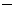1, 42 >, which equals v = < 3, 2 >

I like to remember the formulaas "terminal minus initial". We do it for the q's and the p's!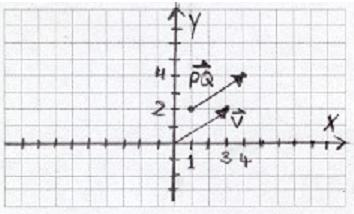Please note that we moved the vector PQ to standard position without changing its magnitude and direction.

Example 2:

Find the component form of the vector with initial point P at (1,3) and terminal point Q at (6, 5) .

Given vector v =and points Pand Q, we can calculate

v = <6(1), 5(3) >, which equals v = <5, 8 >.

I like to remember the formulaas "terminal minus initial". We do it for the q's and the p's!

Example 3:

Calculate the EXACT magnitude of v = < 3, 2 >.

Given ||v|| =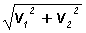, we find ||v|| =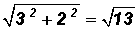Example 4:

Calculate the EXACT magnitude of v = < 0,7 >.

Given ||v|| =, we find ||v|| =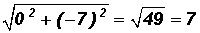Example 5:

Calculate the EXACT magnitude of v = <4,3 >.

Given ||v|| =, we find ||v|| =Example 6:

Calculate the EXACT magnitude of v = < 8, 0 >.

Given ||v|| =, we find ||v|| =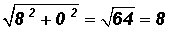Example 7:

Find the direction angle of vector.

Let's look at a picture of the vector and its direction angle. Remember that a direction angle is positive and measured from the positive x-axis to the vector in standard position as shown below!From earlier work we know that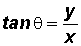, therefore, we find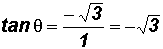andLooking at the graph of the vector, we know that we are required to find a positive angle whose terminal side is in Quadrant IV.

We will use the Reference Angle of60o which is 60o to find that the direction angle must equal 300o.

Example 8:

Find the direction angle of vector.

Let's look at a picture of the vector and its direction angle.Remember that a direction angle is positive and measured from the positive x-axis to the vector in standard position as shown below!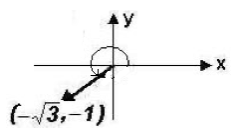Knowing that, we can findandLooking at the graph of the vector, we know that we are required to find a positive angle whose terminal side is in Quadrant IV. We will use the Reference Angle of 30o which is 30o to find that the direction angle must equal 210o.

Example 9:

Let v = <2, 5 > and w = < 3, 4 >.  Find v + w.

v + w = <2, 5 > + < 3, 4 >

= <2 + 3, 5 + 4>

= < 1, 9>

Example 10:

Let v = <2, 5 > and w = < 3, 4 >.  Find the following:

(a)vv = (1)v

= (1)<2, 5 >

= < (1)(2), (1)(5) >

= <2,5>

(b)  2v

2v = 2<2, 5 >

= <4, 10 >

(c)  wv

wv = w + (1)v (Adding the negative of vector v!)

= < 3, 4 > +  (1)<2, 5 >

= < 3, 4 > + < 2,5 >

= < 5,1 >

(d)  v + 2w

v + 2w = <2, 5 > + 2< 3, 4 >

= < 4, 13 >